# NCERT Solutions for Class 9 Maths Chapter 9 Exercise 9.1 – Areas of Parallelograms And Triangles

Download NCERT Solutions for Class 9 Maths Chapter 9 Exercise 9.1 – Areas of Parallelograms And Triangles. This Exercise contains 7 questions, for which detailed answers have been provided in this note. In case you are looking at studying the remaining Exercise for Class 9 for Maths NCERT solutions for Chapter 9 or other Chapters, you can click the link at the end of this Note.

### NCERT Solutions for Class 9 Maths Chapter 9 Exercise 9.1 – Areas of Parallelograms And Triangles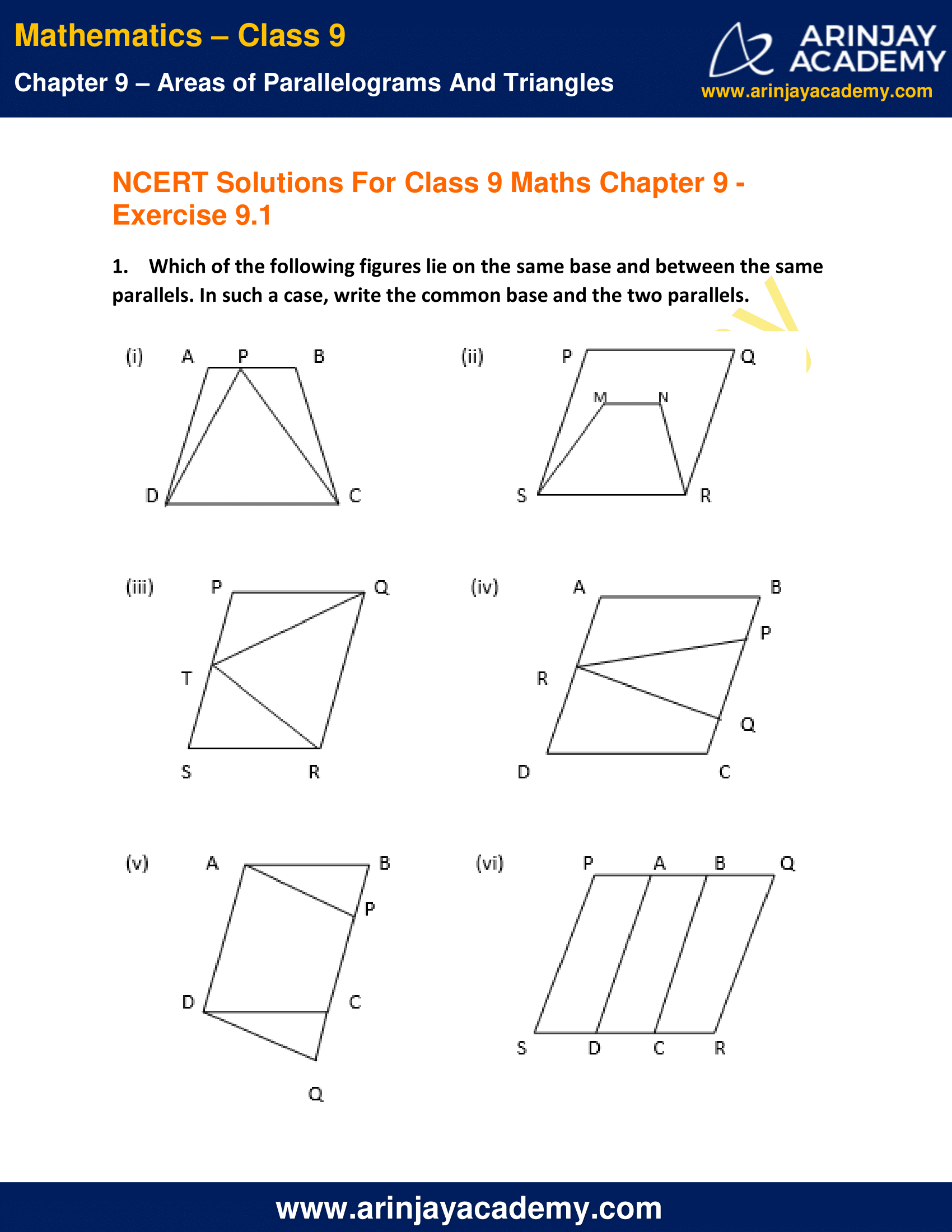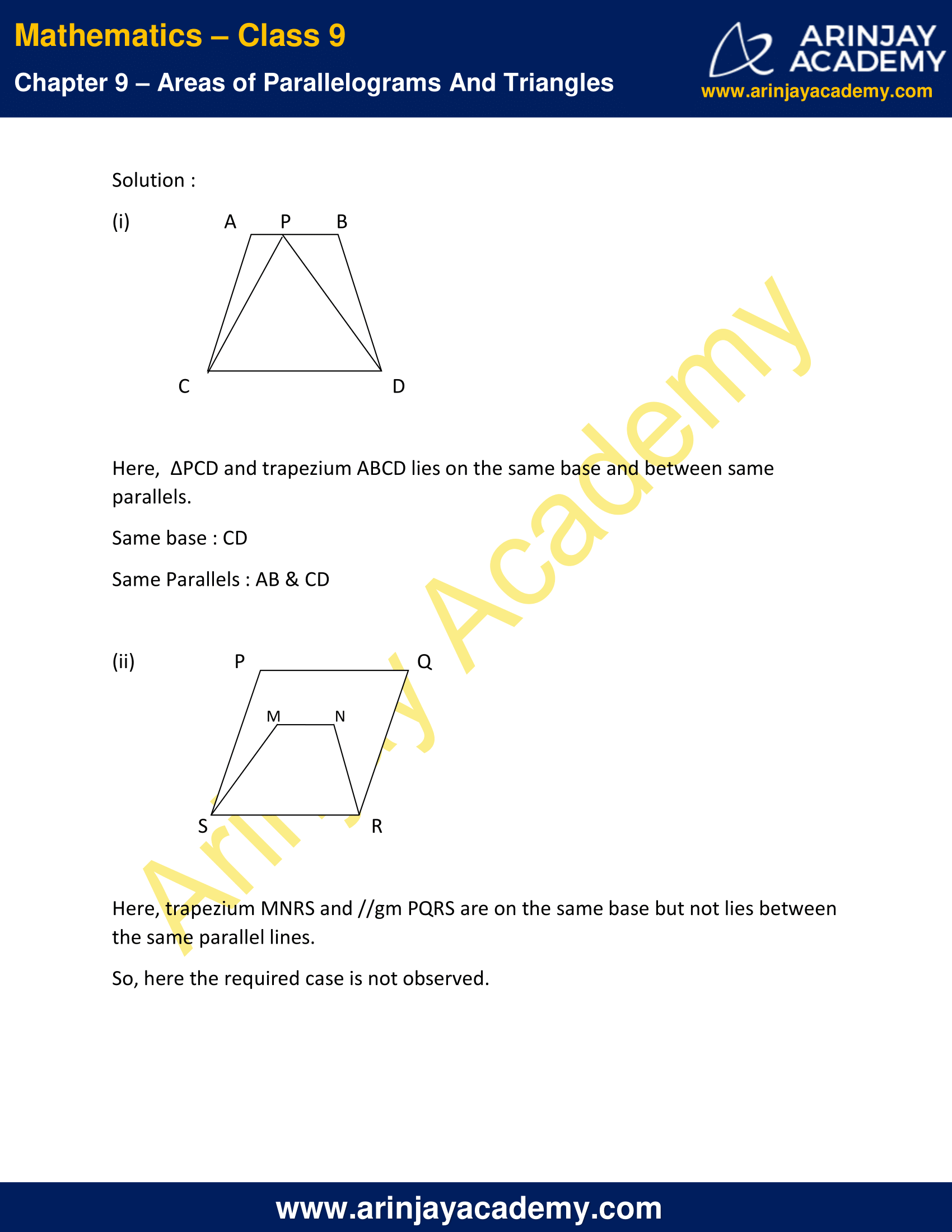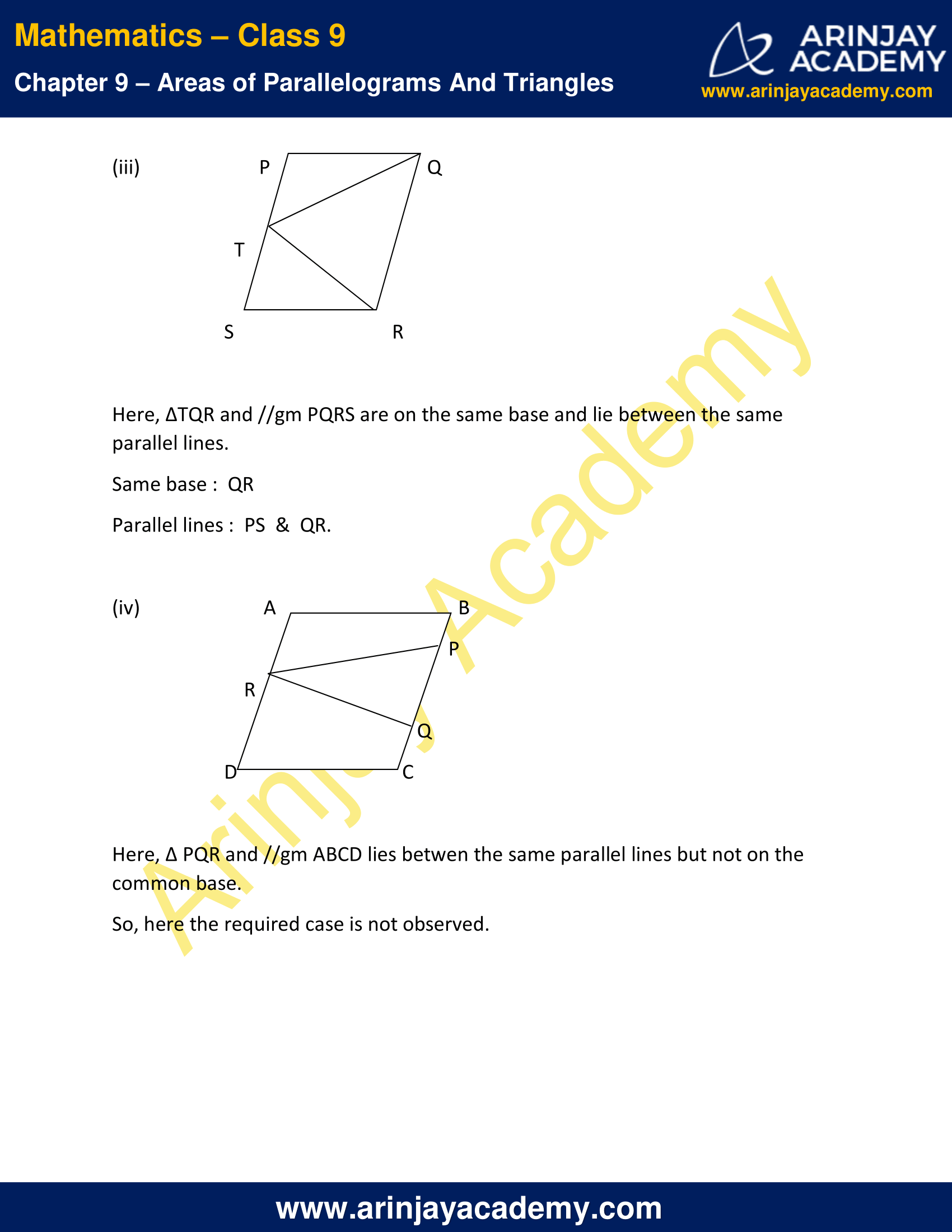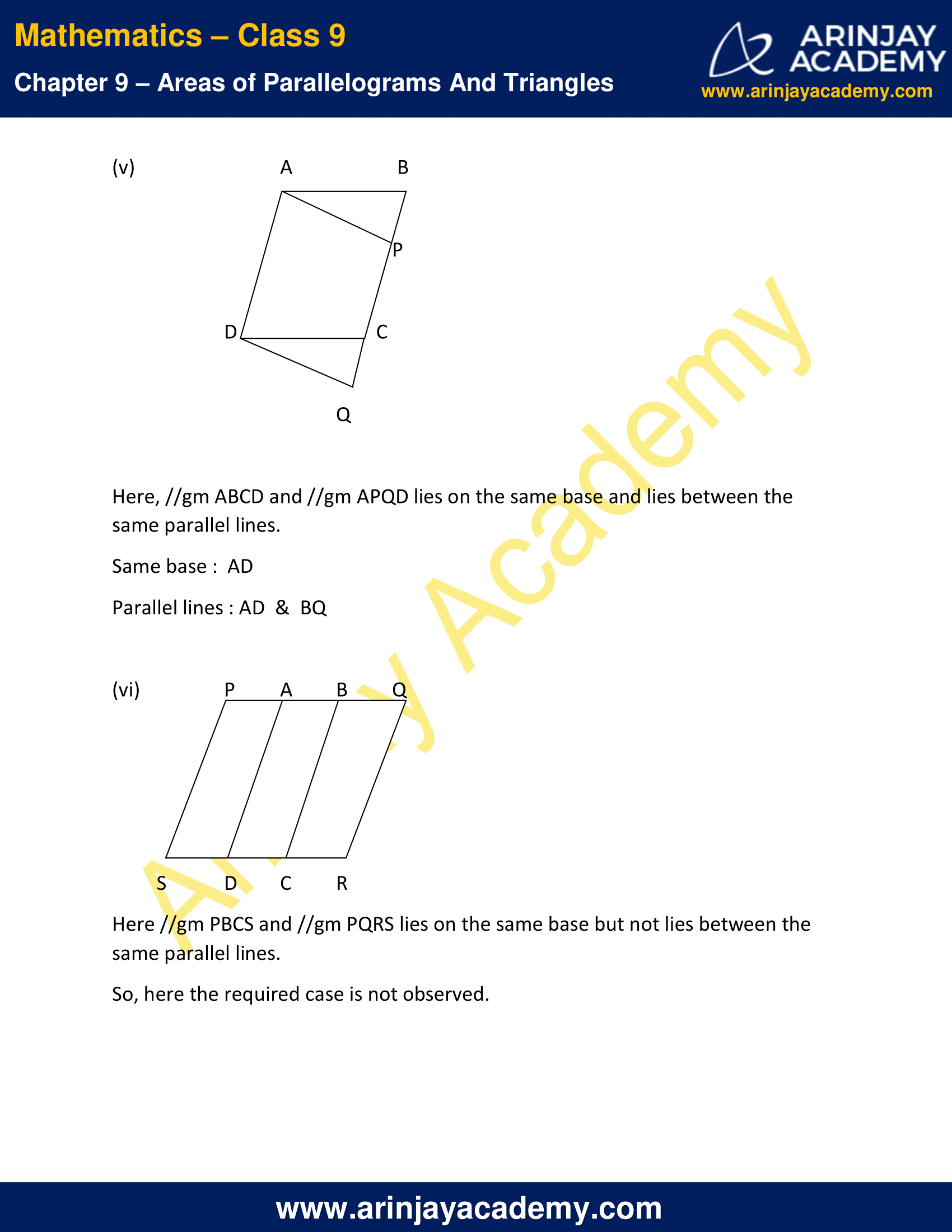NCERT Solutions for Class 9 Maths Chapter 9 Exercise 9.1 – Areas of Parallelograms And Triangles

1. Which of the following figures lie on the same base and between the same parallels. In such a case, write the common base and the two parallels.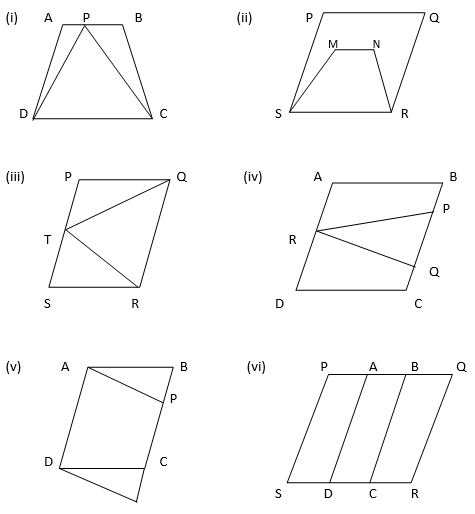Solution: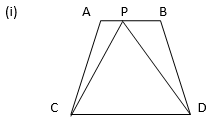Here,  ∆PCD and trapezium ABCD lies on the same base and between same parallels.
Same base : CD
Same Parallels : AB & CD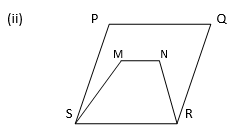Here, trapezium MNRS and //gm PQRS are on the same base but not lies between the same parallel lines.
So, here the required case is not observed.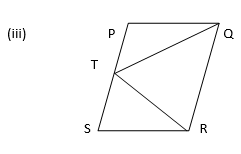Here, ∆TQR and //gm PQRS are on the same base and lie between the same parallel lines.
Same base :  QR
Parallel lines :  PS  &  QR.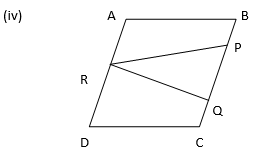Here, ∆ PQR and //gm ABCD lies betwen the same parallel lines but not on the common base.
So, here the required case is not observed.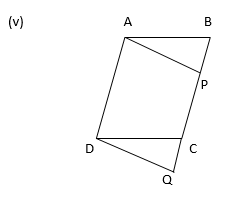Here, //gm ABCD and //gm APQD lies on the same base and lies between the same parallel lines.
Same base :  AD
Parallel lines : AD  &  BQ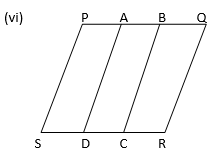Here //gm PBCS and //gm PQRS lies on the same base but not lies between the same parallel lines.
So, here the required case is not observed.

NCERT Solutions for Class 9 Maths Chapter 9 Exercise 9.1 – Areas of Parallelograms And Triangles, has been designed by the NCERT to test the knowledge of the student on the topic – Figures on the Same Base and Between the Same Parallels

The next Exercise for NCERT Solutions for Class 9 Maths Chapter 9 Exercise 9.2 – Areas of Parallelograms And Triangles can be accessed by clicking here.

Download NCERT Solutions for Class 9 Maths Chapter 9 Exercise 9.1 – Areas of Parallelograms And Triangles